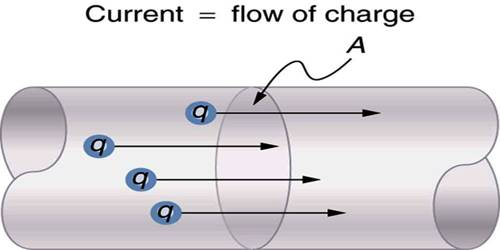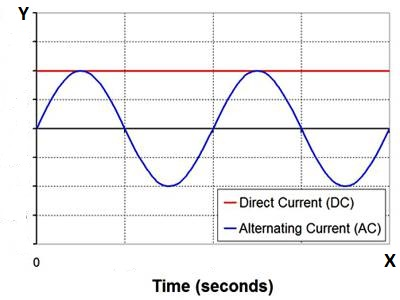Physics

# CurrentCurrent

A flow of charge is called current. But in general meaning, a flow of electrons is current. Electrons remain outside the nucleus and protons remain inside the nucleus. It is easier to flow electrons, the protons, so we say the flow of electrons as current. From the generating center of electricity, electrons do not move like ants from one place to another place. As the bricks stacked side by side and if one brick is pushed then all the bricks fall. Here last brick does not come to the place of first brick. Similarly, electrons do not come from one place to another place. Electrons, arranged in a conductor, move very fast from one place to another place by vibrations.

So, current is a flow of electrical charge carriers, usually electrons, or electron-deficient atoms.

Now we will see how many types of current flow is there. If a resistor is connected across the two terminals of a battery, then current will flow through the resistor. This current has a particular direction. Current originating from the positive terminal reaches to the negative terminal through the resistor. Besides, the value of current remains constant and the direction does not change. This type of current is called D.C. current. If the current flowing through the resistor or any part of this circuit changes direction at a regular interval, i.e., the time for which it flows in one direction, the same current flows in the opposite direction for the same duration, them this type of current is called alternating current. Further, the value of D.C. current remains constant as long as the current flows in the circuit. But the alternating current changes a maximum and a minimum value at a fixed time interval. At a fixed time interval alternating current repeats itself. This fixed time is called the time period. Current remains half of the time period in the positive direction and the rest half in the negative direction i.e., current repeats itself after one time period. In a constant magnetic field if a closed coil rotates with uniform angular velocity, then this type of electromotive force is produced in the coil and we get alternating current.Mathematically flow of charges in unit time is called current, i.e., if Q amount of charges flow in a conductor for time ‘t’, then current I = Q/t (amp). A.C and D.C current have been shown in the graph. The equation of current is, I = I0 sin ωt, here, I0 = peak value of current, ω = angular frequency.